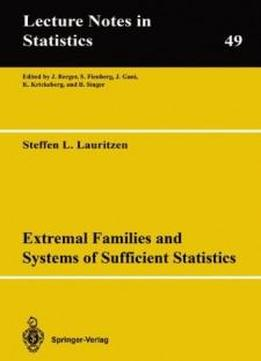# Extremal Families And Systems Of Sufficient Statistics (lecture Notes In Statistics) by Steffen L. Lauritzen / 1988 / English / PDF

The pOint of view behind the present work is that the connection between a statistical model and a statistical analysis-is a dua­ lity (in a vague sense). In usual textbooks on mathematical statistics it is often so that the statistical model is given in advance and then various in­ ference principles are applied to deduce the statistical ana­ lysis to be performed. It is however possible to reverse the above procedure: given that one wants to perform a certain statistical analysis, how can this be expressed in terms of a statistical model? In that sense we think of the statistical analysis and the stati­ stical model as two ways of expressing the same phenomenon, rather than thinking of the model as representing an idealisation of "truth" and the statistical analysis as a method of revealing that truth to the scientist. It is not the aim of the present work to solve the problem of giving the correct-anq final mathematical description of the quite complicated relation between model and analysis. We have rather restricted ourselves to describe a particular aspect of this, formulate it in mathematical terms, and then tried to make a rigorous and consequent investigation of that mathematical struc­ ture.

The pOint of view behind the present work is that the connection between a statistical model and a statistical analysis-is a dua­ lity (in a vague sense). In usual textbooks on mathematical statistics it is often so that the statistical model is given in advance and then various in­ ference principles are applied to deduce the statistical ana­ lysis to be performed. It is however possible to reverse the above procedure: given that one wants to perform a certain statistical analysis, how can this be expressed in terms of a statistical model? In that sense we think of the statistical analysis and the stati­ stical model as two ways of expressing the same phenomenon, rather than thinking of the model as representing an idealisation of "truth" and the statistical analysis as a method of revealing that truth to the scientist. It is not the aim of the present work to solve the problem of giving the correct-anq final mathematical description of the quite complicated relation between model and analysis. We have rather restricted ourselves to describe a particular aspect of this, formulate it in mathematical terms, and then tried to make a rigorous and consequent investigation of that mathematical struc­ ture.

views: 550